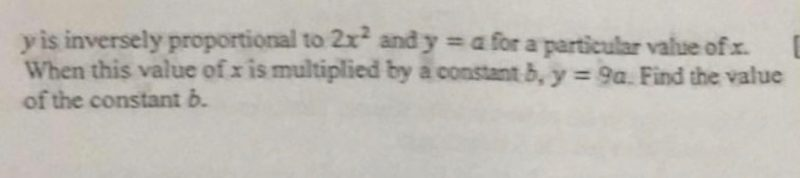QuestionOnce again, not a teacher but trying this out for fun.

Inversely proportional means

y=k/(2x2)

For a particular x value, y = a

This means that :

a = k/(2x2)            — (1)

When x becomes bx, y becomes 9a

9a = k/(2b2x2)      — (2)

Since a = k/(2x2), putting (1) into (2) yields

9 [k/(2x2)] = k/(2b2x2)

9 [k/(2x2)] = k/(2b2x2)

9 = 1/ b2

Square root both sides

3 = 1/b

3b = 1

b = 1/3 !!!

Crap is secondary 2 maths becoming so difficult. i was thinking this is more like sec 4 maths liao!!!

0 Replies 0 Likes